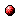# A note on the characteristic equation in the max-plus algebra

Reference:
B. De Schutter and B. De Moor, "A note on the characteristic equation in the max-plus algebra," Linear Algebra and Its Applications, vol. 261, no. 1-3, pp. 237-250, Aug. 1997.

Abstract:
In this paper we discuss the characteristic equation of a matrix in the max-plus algebra. In their Linear Algebra and Its Applications paper (vol. 101, pp. 87-108, 1988) Olsder and Roos have used a transformation between the max-plus algebra and linear algebra to show that the Cayley-Hamilton theorem also holds in the max-plus algebra. We show that the derivation of Olsder and Roos is not entirely correct and we give the correct formulas for the coefficients of this alternative version of the max-algebraic characteristic equation. We also give a counterexample for a conjecture of Olsder in which he states necessary and sufficient conditions for the coefficients of the max-algebraic characteristic equation.

Downloads:Online version of the paperCorresponding technical report: pdf file (171 KB)
Note: More information on the pdf file format mentioned above can be found here.

Bibtex entry:

@article{DeSDeM:96-30,
author={B. {D}e Schutter and B. {D}e Moor},
title={A note on the characteristic equation in the max-plus algebra},
journal={Linear Algebra and Its Applications},
volume={261},
number={1--3},
pages={237--250},
month=aug,
year={1997},
doi={10.1016/S0024-3795(96)00407-7}
}

Go to the publications overview page.

This page is maintained by Bart De Schutter. Last update: August 12, 2020.